# State bank of india stock price analysis and forcasting of 2023

## How to collect sbi historical price?

Analyzing and forecasting the stock price of the State Bank of India (SBI) involves examining historical price data, conducting data analysis, and applying forecasting techniques. Here's a step-by-step guide on how to perform SBI stock price analysis and forecasting using Python:

Import Libraries:
Start by importing the necessary Python libraries for data manipulation, analysis, and visualization. Commonly used libraries include pandas, numpy, matplotlib, yfinance for fetching historical data, and libraries for time series forecasting, such as stats models or Prophet:

Python
Copy code
import pandas as pd
import numpy as np
import matplotlib.pyplot as plt
import yfinance as yf
from statsmodels.tsa.holtwinters import ExponentialSmoothing
Data Retrieval:
Use the yfinance library or other financial data sources to fetch historical price data for SBI's stock. Specify the start and end dates for the data you want to analyze:

Python
Copy code
Data Exploration:
Explore the fetched data to understand its structure and contents. Use functions like head(), tail(), describe(), and info() to inspect the dataset:

Python
Copy code
Data Visualization:
Create visualizations to analyze the historical performance of SBI's stock. Common visualizations include line charts to visualize price movements:

Python
Copy code
plt.figure(figsize=(12, 6))
plt.title('SBI Stock Price')
plt.xlabel('Date')
plt.ylabel('Price')
plt.legend()
plt.show()
Time Series Analysis:
Conduct time series analysis to understand trends, seasonality, and potential stationarity in the data. Use tools like autocorrelation and partial autocorrelation plots to identify the order of differencing for stationarity.

Data Preprocessing:
Prepare the data for forecasting by splitting it into training and testing sets, handling missing values, and applying any necessary transformations.

Forecasting Model Selection:
Choose an appropriate forecasting model. Popular models for time series forecasting include ARIMA (AutoRegressive Integrated Moving Average), Exponential Smoothing, and Prophet.

For example, to apply Exponential Smoothing:

Python
Copy code
results = model.fit()
forecast = results.forecast(steps=12)  # Forecast for the next 12 months
Model Evaluation:
Evaluate the forecasting model's performance using appropriate metrics such as Mean Absolute Error (MAE), Mean Squared Error (MSE), and Root Mean Squared Error (RMSE). Compare the forecasted values to the actual values in the testing set.

Visualization of Forecast:
Visualize the forecasted stock prices alongside the actual prices to assess the model's accuracy.

Keep your forecasting model up to date with the latest data and monitor its performance over time. Adjust the model as needed to improve accuracy.

Risk Management and Decision Making:
Based on your analysis and forecasting, formulate investment strategies, set risk management parameters, and make informed investment decisions regarding SBI's stock.

Please note that forecasting stock prices is inherently uncertain, and predictions should be used cautiously for investment decisions. The choice of forecasting model and parameters may require tuning, and additional factors such as market news, economic indicators, and company-specific events should also be considered when making investment decisions.

!pip install yfinance

### How to install sbi data from yahoo in python?

import yfinance as yf
import numpy as np
import os
import random
import torch
import torch.optim as optim
import torch.nn as nn
from torch.utils.data import (Dataset, TensorDataset,
from collections import OrderedDict
from sklearn.metrics import mean_squared_error
import pandas as pd
from statsmodels.tsa.seasonal import seasonal_decompose
start='2010-01-01',
end='2021-09-08',
progress=False)
df.tail()
df.describe()
df = df.resample('M').last()
WINDOW_SIZE = 12
df['rolling_mean'] = df.price.rolling(window=WINDOW_SIZE).mean()
df['rolling_std'] = df.price.rolling(window=WINDOW_SIZE).std()
df.plot(title='State Bank Stock Price')
`<matplotlib.axes._subplots.AxesSubplot at 0x7f92720ec250>`

### How to plot sbi historical price?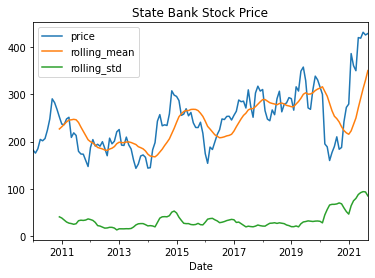### How to plot sbi Multiplicative Decomposition ?

decomposition_results = seasonal_decompose(df.price,
model='multiplicative')
decomposition_results.plot() \
.suptitle('Multiplicative Decomposition',
fontsize=18);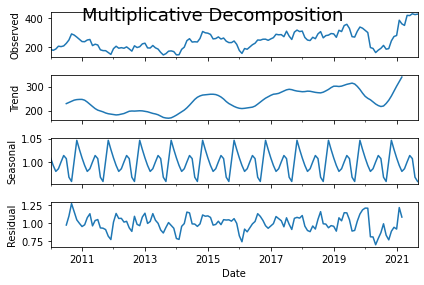### How to forcast sbi stock price in 2022?

import pandas as pd
import seaborn as sns
from fbprophet import Prophet
train_indices = df.ds.apply(lambda x: x.year) < 2021
df_train = df.loc[train_indices].dropna()
df_test = df.loc[~train_indices].reset_index(drop=True)
model_prophet.fit(df_train)
df_future = model_prophet.make_future_dataframe(periods=365)
df_pred = model_prophet.predict(df_future)
model_prophet.plot(df_pred)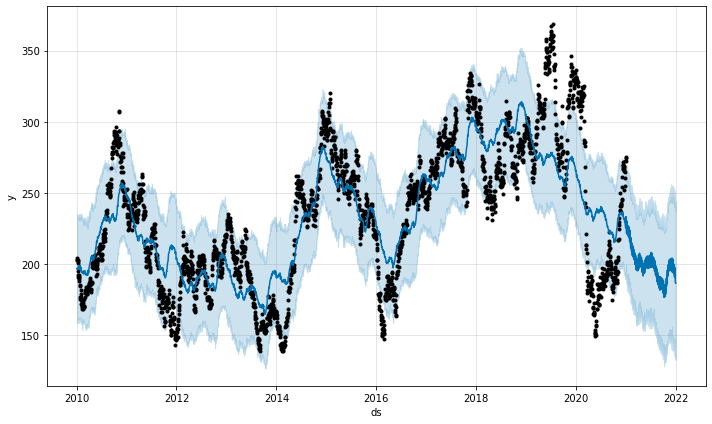### How to forcast sbi seasonal stock price in python?

model_prophet.plot_components(df_pred)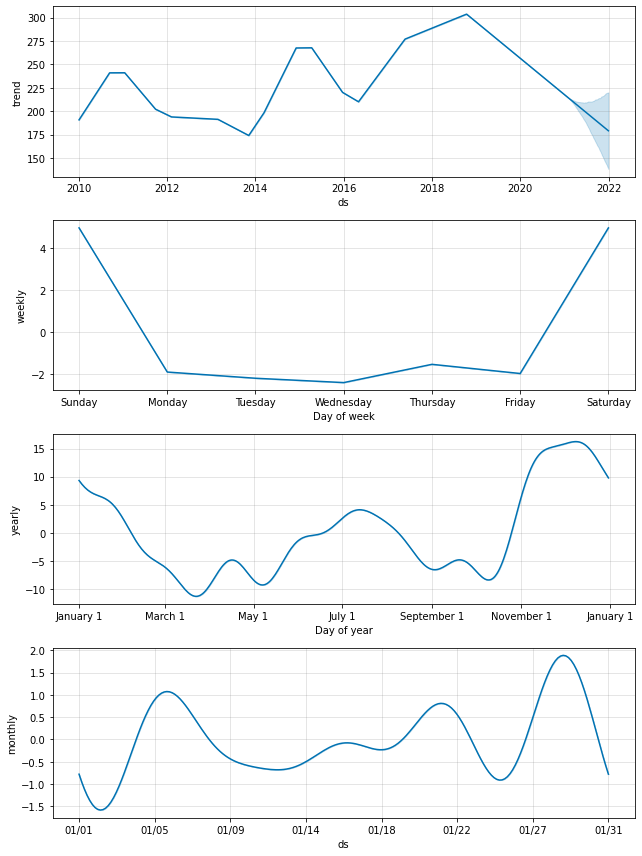selected_columns = ['ds''yhat_lower''yhat_upper''yhat']
df_pred = df_pred.loc[:, selected_columns].reset_index(drop=True)
df_test = df_test.merge(df_pred, on=['ds'], how='left')
df_test.ds = pd.to_datetime(df_test.ds)
df_test.set_index('ds', inplace=True)
import matplotlib.pyplot as plt
fig, ax = plt.subplots(11)
ax = sns.lineplot(data=df_test[['y''yhat_lower''yhat_upper','yhat']])
ax.fill_between(df_test.index,
df_test.yhat_lower,
df_test.yhat_upper,
alpha=0.3)
ax.set(title='Stock Price - actual vs. predicted',
xlabel='Date',
ylabel='Stock Price ')

### How to predict sbi stock price in python?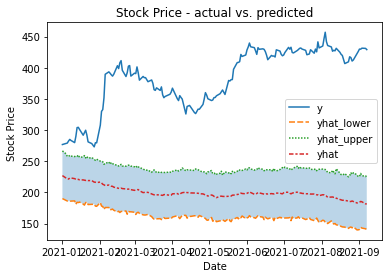import pandas as pd
from statsmodels.graphics.tsaplots import plot_acf, plot_pacf
indices = ['Test Statistic''p-value',
'# of Lags Used''# of Observations Used']
results[f'Critical Value ({key})'] = value
return results

### How to find sbi adf test in python?

Test Statistic -2.152640 p-value 0.223881 # of Lags Used 17.000000 # of Observations Used 2862.000000 Critical Value (1%) -3.432637 Critical Value (5%) -2.862550 Critical Value (10%) -2.567308 dtype: float64

### How to forcast sbi stock price kpss test in python?

def kpss_test(xh0_type='c'):
indices = ['Test Statistic''p-value''# of Lags']
kpss_test = kpss(x, regression=h0_type)
results = pd.Series(kpss_test[0:3], index=indices)
for key, value in kpss_test.items():
results[f'Critical Value ({key})'] = value
return results
kpss_test(df.y)
Test Statistic 3.723934 p-value 0.010000 # of Lags 28.000000 Critical Value (10%) 0.347000 Critical Value (5%) 0.463000 Critical Value (2.5%) 0.574000 Critical Value (1%) 0.739000 dtype: float64
N_LAGS = 40
SIGNIFICANCE_LEVEL = 0.05
fig, ax = plt.subplots(21, figsize=(1612), sharex=True)
plot_acf(df.y, ax=ax, lags=N_LAGS,
alpha=SIGNIFICANCE_LEVEL)
plot_pacf(df.y, ax=ax, lags=N_LAGS,
alpha=SIGNIFICANCE_LEVEL)